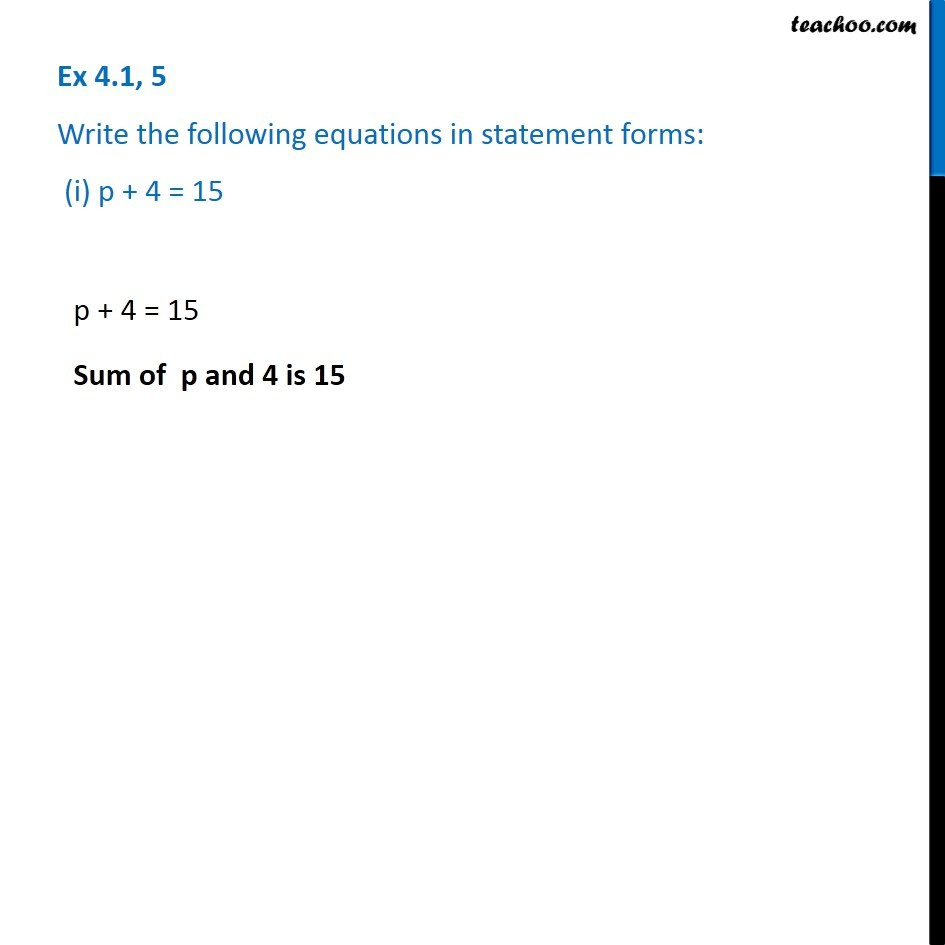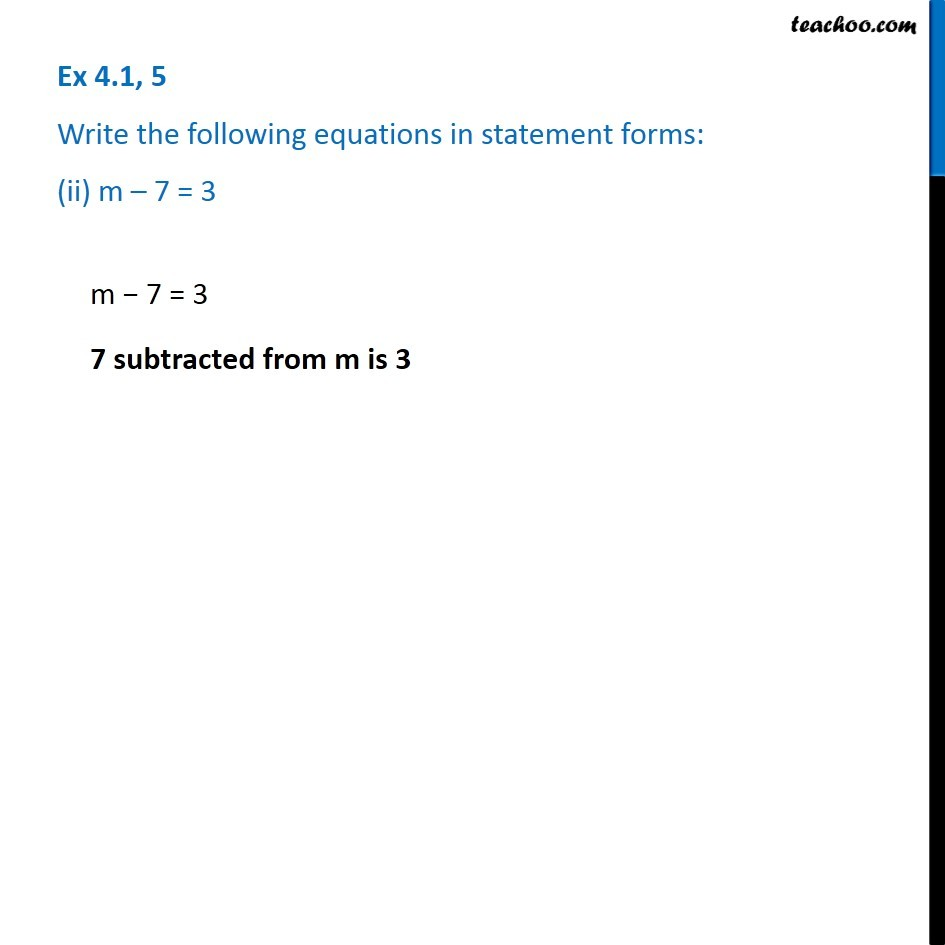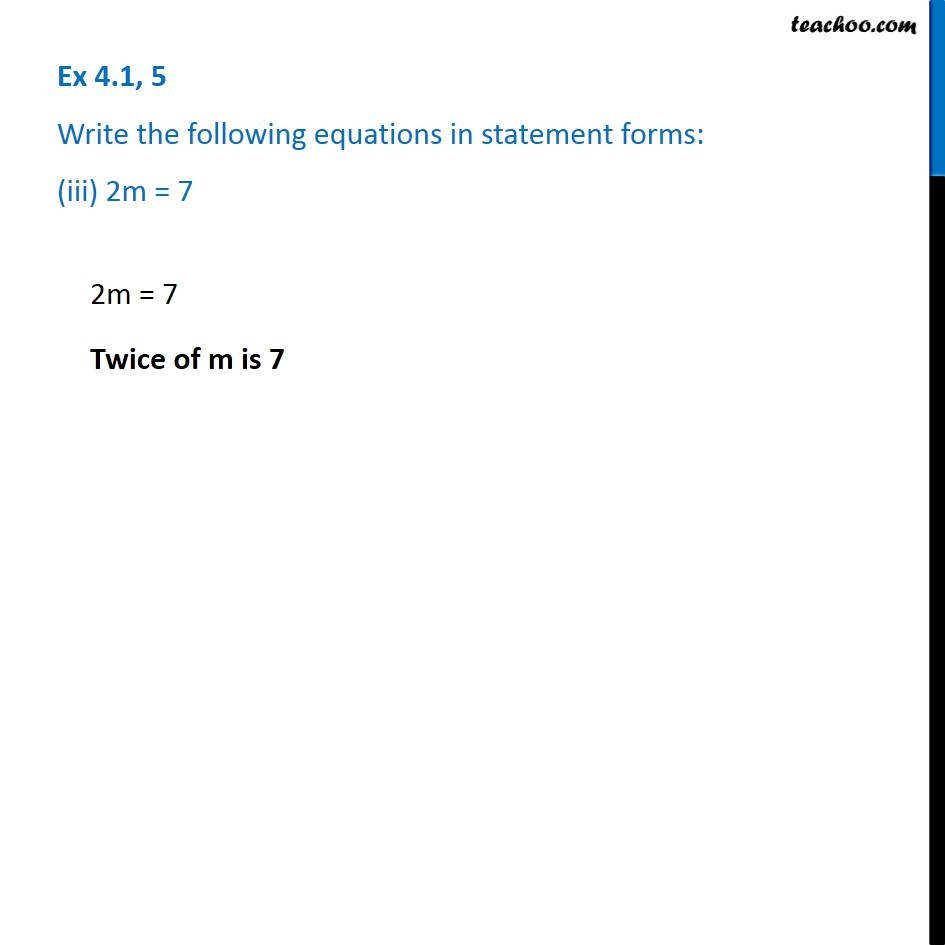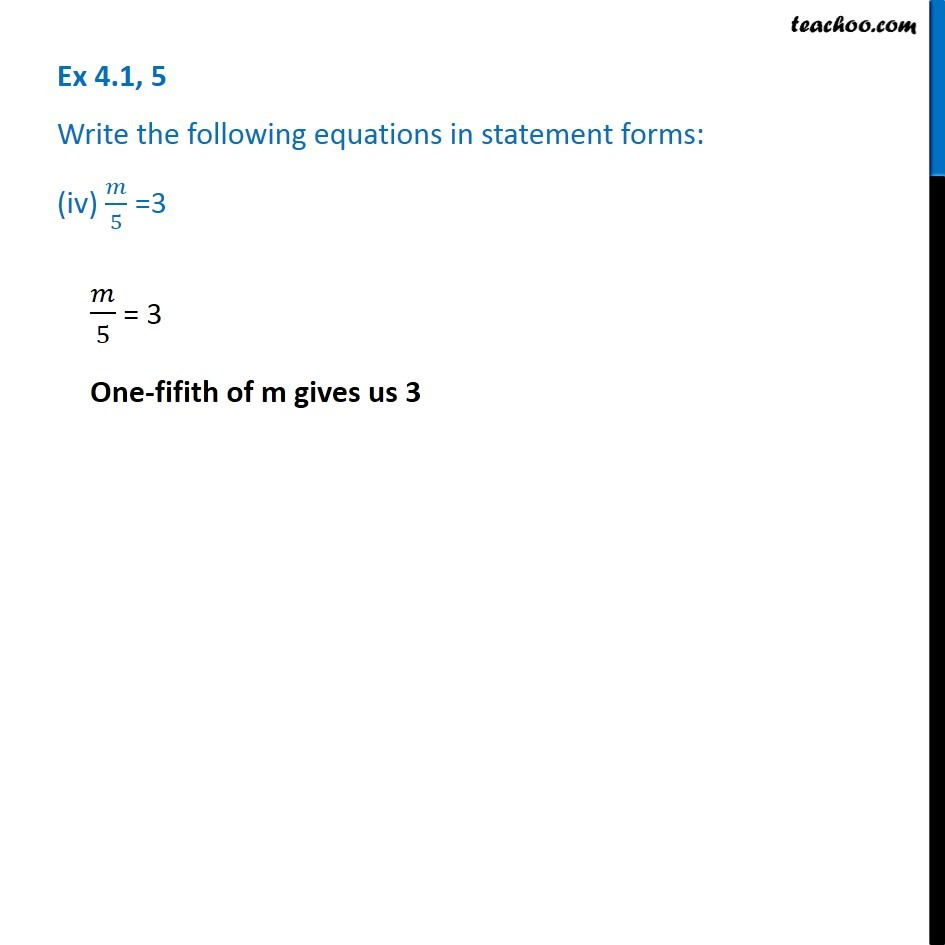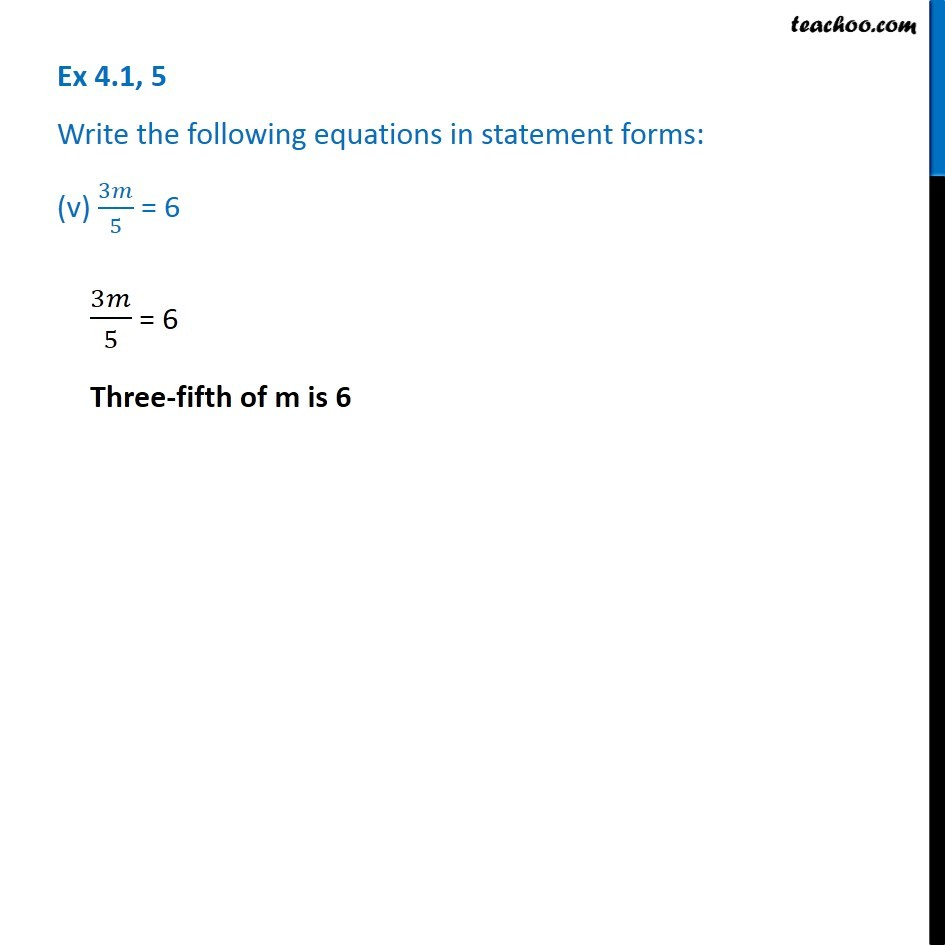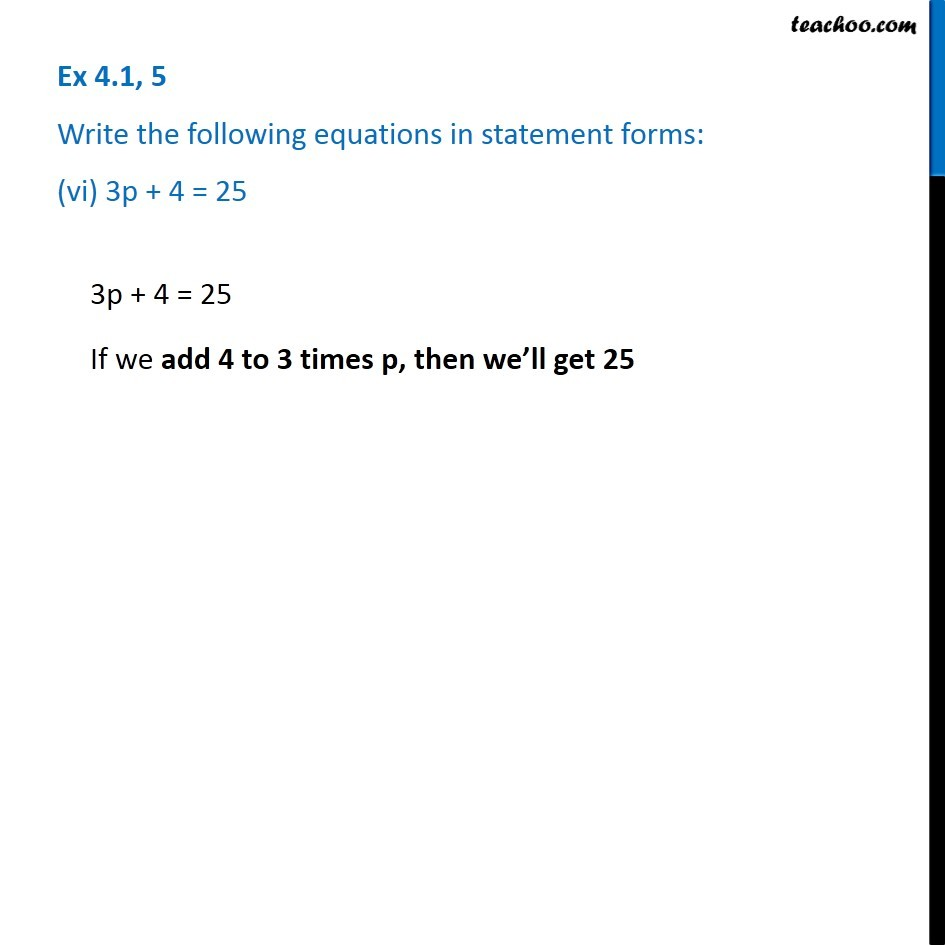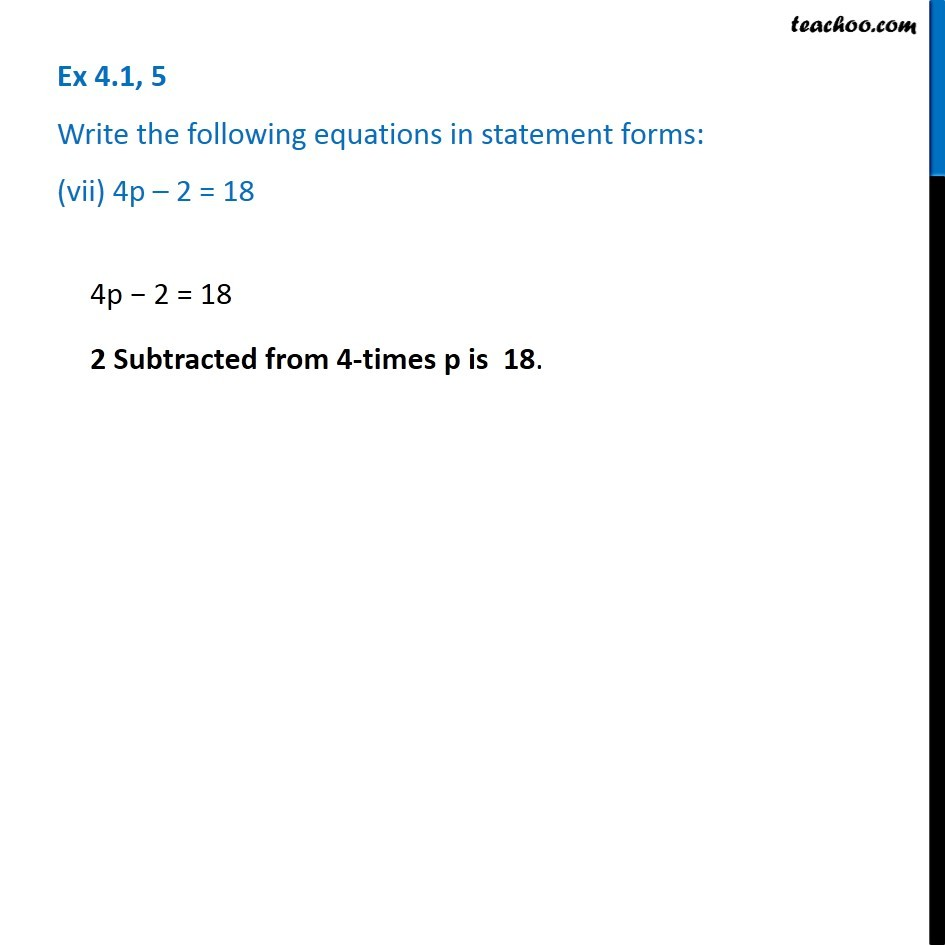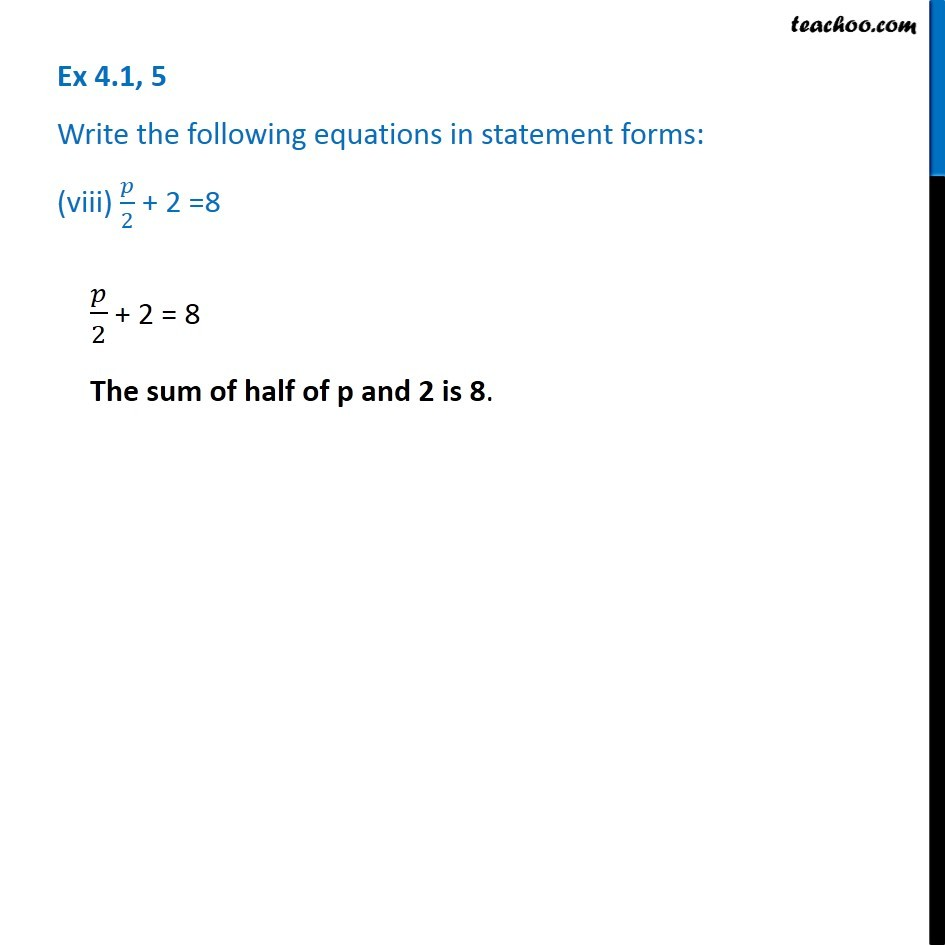1. Chapter 4 Class 7 Simple Equations
2. Serial order wise
3. Ex 4.1

Transcript

Ex 4.1, 5 Write the following equations in statement forms: (i) p + 4 = 15p + 4 = 15 Sum of p and 4 is 15 Ex 4.1, 5 Write the following equations in statement forms: (ii) m – 7 = 3 m − 7 = 3 7 subtracted from m is 3 Ex 4.1, 5 Write the following equations in statement forms: (iii) 2m = 7 2m = 7 Twice of m is 7 Ex 4.1, 5 Write the following equations in statement forms: (iv) 𝑚/5 =3𝑚/5 = 3 One-fifith of m gives us 3 Ex 4.1, 5 Write the following equations in statement forms: (v) 3𝑚/5 = 6 Ex 4.1, 5 Write the following equations in statement forms: (vi) 3p + 4 = 25 3p + 4 = 25 If we add 4 to 3 times p, then we’ll get 25 Ex 4.1, 5 Write the following equations in statement forms: (vii) 4p – 2 = 18 4p − 2 = 18 2 Subtracted from 4-times p is 18Ex 4.1, 5 Write the following equations in statement forms: (viii) 𝑝/2 + 2 =8𝑝/2 + 2 = 8 The sum of half of p and 2 is 8.

Ex 4.1